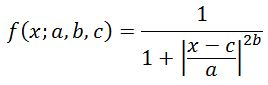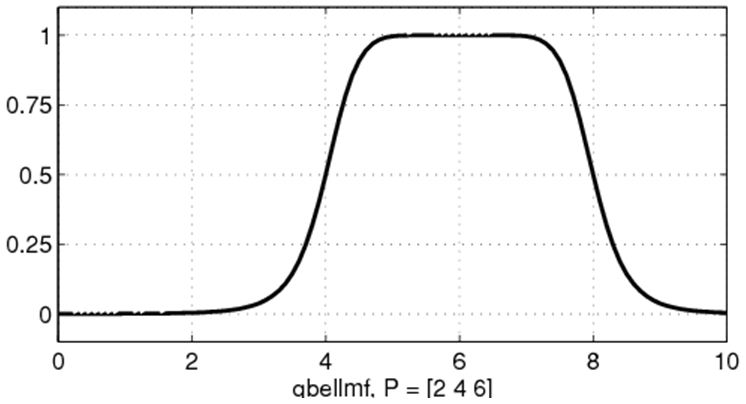### Introduction

The generalized bell function depends on three parameters a, b and c as given by:Each of these parameters has a physical meaning: c determines the centre of the corresponding membership function; a is the half width; and b (together with a) controls the slopes at the crossover points. Figure below shows these concepts.Figure 2: The effects of changing parameters in bell function: (a) changing 'a'; (b) changing 'b'; (c) changing 'c'; (d) changing 'a' and 'b' simultaneously but keeping their ratio constant.

### Matlab

In Matlab, this Bell-shaped function is defined as:

`y=dbellmf(x,params)`

Example:

`x=0:0.1:10;y=gbellmf(x,[2 4 6]);plot(x,y)xlabel('gbellmf, P=[2 4 6]')`### References & Resources

• Matlab - http://www.mathworks.co.uk/help/toolbox/fuzzy/gbellmf.html